﻿ 基于像素缩放残差的块效应的图像局部变形检测方法 Image Local Deformation Detection Method Based on Block Effect of Pixel Scaling Residual

Computer Science and Application
Vol. 09  No. 07 ( 2019 ), Article ID: 31298 , 8 pages
10.12677/CSA.2019.97145

Image Local Deformation Detection Method Based on Block Effect of Pixel Scaling Residual

Ruikun Wang

School of Computer Science and Technology, Tianjin Polytechnic University, Tianjin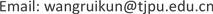Received: Jun. 30th, 2019; accepted: Jul. 10th, 2019; published: Jul. 17th, 2019ABSTRACT

The use of tampered images in illegal ways will infringe citizens’ right to know and portrait. Local distortion of images is often used in image thinning operations. This paper proposes an improved method to detect local distortion of JPEG compressed images, which can better detect the location of local distortion of images. Firstly, the residual image of the detected image is extracted by one-pixel scaling method, and then the block-effect mesh with a period of 8 is extracted from the residual image. Then, the location of the local distortion of the image is detected according to the inconsistency of the block-effect mesh. In detecting the inconsistency of the block-effect, we use new features. Experiments show that the proposed method improves the detection results of the original block effect.

Keywords:Image Scaling, BAG, JPEG, Local Image Deformation, Image ForensicsCopyright © 2019 by author(s) and Hans Publishers Inc.1. 引言

Bharati  等人提出了一种新的使用有监督的深度玻尔兹曼机的算法，在人脸图片中选取了4个部分学习特征，通过使用提出的算法判别人脸图像是修饰过的还是原始图像。该方法并没有检测图像是否发生变形篡改以及定位篡改发生的位置。

Hwang  提出了一种图像局部变形的检测方法，他们通过研究几何变换中的差值模式，提出了一种重新插值的方法检测几何变形，但是这种需要直观观察的方法在图像局部几何变形中的检测效果并不是很理想。对于压缩图像的篡改的检测效果更是不好。图1显示了使用Hwang的方法检测图像局部集合变相的结果。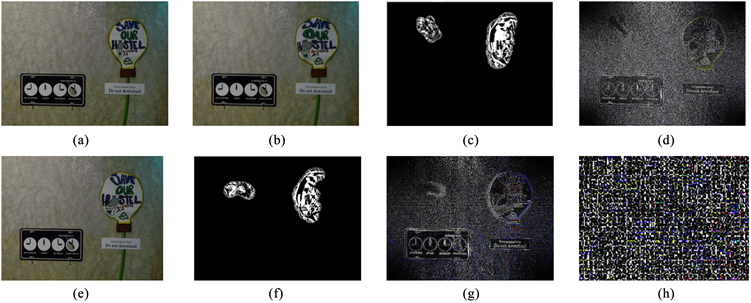Figure 1. Detecting local deformation of image after scaling of 1 pixel

JPEG在压缩的过程中是对图像进行分块处理的，对每个压缩块计算其离散余弦变换(DCT)，这种压缩方法使得图像不同块之间的关系发生弱化，块中像素相关性较大而块间的像素相关性变弱，造成快件出现断层，即块效应。块效应常用来检测图像的复制粘贴及拼接操作，人们在对图像进行上述篡改操作时主要考虑将复制或剪切的部分放置在图像的合适位置，并不考虑图像的块效应是否一致。同样，在对图像进行局部变形时会改变变形区域像素的值，使像素发生移动的现象，这种情况破坏了压缩图像的块效应。图2展示了对于压缩未篡改图像的块效应网格以及压缩篡改图像的块效应网格。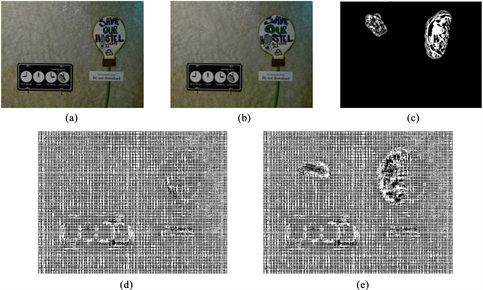Figure 2. Block effect grid for compressed images

2. 提出的方法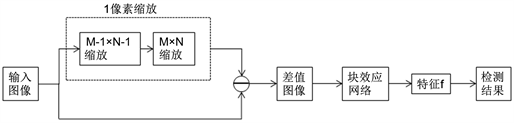Figure 3. Method flow chart

2.1. 缩放残差图像提取

$I\left(x,y\right)\stackrel{\text{STEP1}}{\to }{I}^{\prime }\left({x}^{\prime },{y}^{\prime }\right)\stackrel{\text{STEP2}}{\to }\stackrel{^}{I}\left({x}^{\prime },{y}^{\prime }\right)$ (1)

$\text{step1}=\left\{\begin{array}{c}0\le x\le {N}_{x}-1,x\in z\\ 0\le y\le {N}_{y}-1,y\in z\end{array}$ (2)

$\text{step2}=\left\{\begin{array}{c}0\le {x}^{\prime }\le {N}_{x}-2,x\in z\\ 0\le {y}^{\prime }\le {N}_{y}-2,y\in z\end{array}$ (3)

${K}_{\left(K\right)}=\left\{\begin{array}{l}\left(a+2\right){|x|}^{3}-\left(a+3\right){|x|}^{2}+1,\text{\hspace{0.17em}}\text{for}\text{\hspace{0.17em}}|x|\le 1\\ a{|x|}^{3}-5a{|x|}^{2}+8a|x|-4a,\text{\hspace{0.17em}}\text{for}\text{\hspace{0.17em}}1<|x|<2\\ 0,\text{\hspace{0.17em}}\text{otherwise}\end{array}$ (4)

$g\left(x,y\right)=\underset{l=-1}{\overset{2}{\sum }}\underset{m=-1}{\overset{2}{\sum }}{C}_{i+l,j+m}K\left({k}_{x}\right)K\left({k}_{y}\right)$ (5)

$D\left(x,y\right)=I\left(x,y\right)-\stackrel{^}{I}\left({x}^{\prime },{y}^{\prime }\right)$ (6)

2.2. BAG提取

2.2.1. 提取弱水平线Eh

${e}_{I}\left(x,y\right)=\underset{i=y-16}{\overset{16}{\sum }}d\left(x,i\right)$ (7)

$e\left(x,y\right)={e}_{I}\left(x,y\right)-Mid\left[\left\{{e}_{I}\left(i,y\right)|x-16\le i\le y+16\right\}\right]$ (8)

2.2.2. 从图像Eh中提取具有8周期的行

${g}_{h}\left(x,y\right)=Mid\left[\left\{e\left(i,y\right)|i=x-16,x-8,x,x+8,x+16\right\}\right]$ (9)

$g\left(x,y\right)={g}_{h}\left(x,y\right)+{g}_{v}\left(x,y\right)$ (10)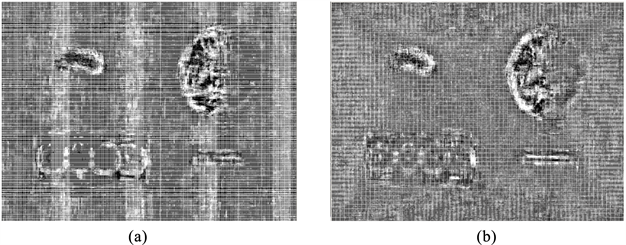Figure 4. Block effect mesh extraction

2.2.3. 计算图像G的特征f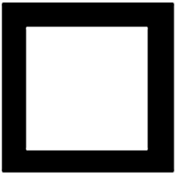(a) Untampered block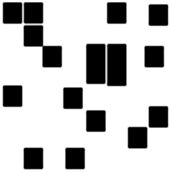(b) Tamper block

Figure 5. 8 × 8 Block effect grid of image

$f=ave\left[\underset{i=2}{\overset{7}{\sum }}\underset{j=2}{\overset{7}{\sum }}a\left(i,j\right)\right]-ave\left[\left\{\underset{i=1}{\overset{8}{\sum }}a\left(x,i\right)|x=1,8\right\}+\left\{a\left(x,1\right)|2\le x\le 7\right\}+\left\{a\left(x,8\right)|2\le x\le 7\right\}\right]+b$ (11)

3. 实验分析

3.1. 特征偏移参数选择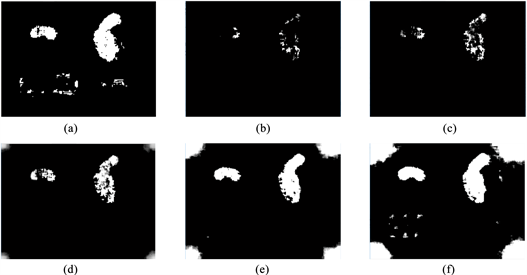Figure 6. Method test results

3.2. 方法实验结果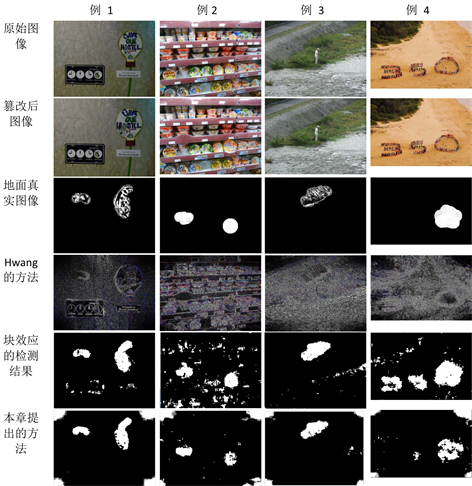Figure 7. Some experimental results are shown

4. 总结

Image Local Deformation Detection Method Based on Block Effect of Pixel Scaling Residual[J]. 计算机科学与应用, 2019, 09(07): 1288-1295. https://doi.org/10.12677/CSA.2019.97145

1. 1. Kee, E. and Farid, H. (2011) A Perceptual Metric for Photo Retouching. Proceedings of the National Academy of Sciences of the United States of America, 108, 19907-19912. https://doi.org/10.1073/pnas.1110747108

2. 2. Bharati, A., Singh, R., Vatsa, M. and Bow-yer, K.W. (2016) Detecting Facial Retouching Using Supervised Deep Learning. IEEE Transactions on Information Forensics and Security, 11, 1903-1913. https://doi.org/10.1109/TIFS.2016.2561898

3. 3. Hwang, M.G., Kim, S.M. and Har, D.H. (2017) A Method of Identifying Digi-tal Images with Geometric Distortion. Australian Journal of Forensic Sciences, 49, 93-105. https://doi.org/10.1080/00450618.2015.1128970

4. 4. Fu, D., Shi, Y.Q. and Su, W. (2007) A Generalized Benford’s Law for JPEG Coefficients and Its Applications in Image Forensics. Conference on Security Steganography and Watermarking of Multimedia Contents, 65051L. https://doi.org/10.1117/12.704723

5. 5. Yang, J., Xie, J., Zhu, G., Kwong, S. and Shi, Y. (2014) An Effective Method for De-tecting Double JPEG Compression With the Same Quantization Matrix. IEEE Transactions on Information Forensics and Security, 9, 1933-1942. https://doi.org/10.1109/TIFS.2014.2359368

6. 6. Bianchi, T. and Piva, A. (2012) Detection of Nonaligned Double JPEG Com-pression Based on Integer Periodicity Maps. IEEE Transactions on Information Forensics and Security, 7, 842-848. https://doi.org/10.1109/TIFS.2011.2170836

7. 7. Pasquini, C., Boato, G. and Perezgonzalez, F. (2014) Multiple JPEG Compres-sion Detection by Means of Benford-Fourier Coefficients. International Workshop on Information Forensics and Security, Atlanta, 3-5 December 2014, 113-118. https://doi.org/10.1109/WIFS.2014.7084313

8. 8. Fan, Z. and De Queiroz, R.L. (2003) Identification of Bitmap Compression History: JPEG Detection and Quantizer Estimation. IEEE Transactions on Image Processing, 12, 230-235. https://doi.org/10.1109/TIP.2002.807361

9. 9. Li, W., Yuan, Y. and Yu, N. (2009) Passive Detection of Doctored JPEG Image via Block Artifact Grid Extraction. Signal Processing, 89, 1821-1829. https://doi.org/10.1016/j.sigpro.2009.03.025

10. 10. Christlein, V., Riess, C., Jordan, J. and Angelopoulou, E. (2012) An Evaluation of Popular Copy-Move Forgery Detection Approaches. IEEE Transactions on Information Forensics and Security, 7, 1841-1854. https://doi.org/10.1109/TIFS.2012.2218597